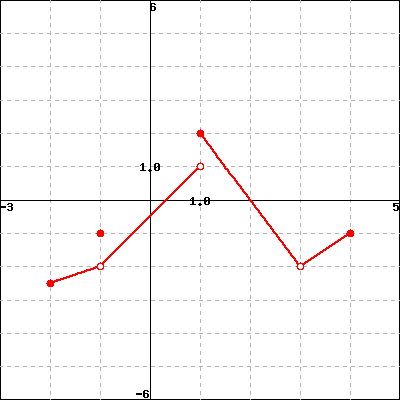Let F be the function below.

If you are having a hard time seeing the picture clearly, click on the picture. It will expand to a larger picture on its own page so that you can inspect it more clearly.Evaluate each of the following expressions.

Note: Enter 'DNE' if the limit does not exist or is not defined.

a) $\displaystyle \lim_{x \to -1^-} F(x)$ =

b) $\displaystyle \lim_{x \to -1^+} F(x)$ =

c) $\displaystyle \lim_{x \to -1} F(x)$ =

d) $F(-1)$ =

e) $\displaystyle \lim_{x \to 1^-} F(x)$ =

f) $\displaystyle \lim_{x \to 1^+} F(x)$ =

g) $\displaystyle \lim_{x \to 1} F(x)$ =

h) $\displaystyle \lim_{x \to 3} F(x)$ =

i) $F(3)$ =

You can earn partial credit on this problem.